# Predicting Stock Prices¶

This project is taken up to demonstrate Machine Learning's ability to predict one of the most challenging problems in financial world - "to predict the unpredictable" - predict the stock price.

In this project, I have used only those techniques which we have studied in Topic 9 of the course with regards to Time Series analysis.

For a use case to show the predictive power of very simple algorithms such as Lasso AND Ridge regressions, I have downloaded the data for a very famous stock in India - "TATA MOTORS".

The link to this data is mentioned here -

https://in.finance.yahoo.com/quote/TATAMOTORS.NS/history?period1=662754600&period2=1544985000&interval=1d&filter=history&frequency=1d

There are few important characteristics which I would like to outline here:

1. We have around 17 years of information (from 02 Jan 1991 till 14 Dec 2018)
1. There are two types of prices given in the data:

a. Closing Prices which do not take into account of any corporate actions in the prices such as declaration of dividends or split of shares effect.

b. Adjusted Closing Prices which do take care of effect of Dividend payments and stock split. [This will be our target variable]

1. There are other information available as well in the data which may not be useful for our analysis point of view.

So, let's dive in.

In :
import pandas as pd
import numpy as np
from fbprophet import Prophet
import matplotlib.pyplot as plt
%matplotlib inline

#setting figure size
from matplotlib.pyplot import rcParams
rcParams['figure.figsize'] = 20,10

#for normalizing data
from sklearn.preprocessing import MinMaxScaler
scaler = MinMaxScaler(feature_range=(0, 1))

import warnings
warnings.filterwarnings('ignore')

In :
# loading basic ML algoriths
from sklearn.linear_model import LinearRegression, RidgeCV, LassoCV
from sklearn.metrics import mean_absolute_error, mean_squared_error
from sklearn.preprocessing import StandardScaler
from sklearn.model_selection import TimeSeriesSplit, cross_val_score

# few powerful algorithms as well which we will see later dont perform well compared to basic algorithms
import xgboost
import lightgbm

In :
# load the downloaded data
import os
os.chdir('C:\\Users\\Abhik\\mlcourse.ai\\mlcourse.ai-master\\data')

In :
# load the data into pandas dataframe

Out:
Date Open High Low Close Adj Close Volume
0 1991-01-02 20.9596 21.857901 20.9596 21.857901 4.031160 0.0
1 1991-01-03 20.9596 21.857901 20.9596 21.857901 4.031160 0.0
2 1991-01-04 NaN NaN NaN NaN NaN NaN
3 1991-01-07 20.3608 21.259001 20.0613 21.109301 3.893099 0.0
4 1991-01-08 NaN NaN NaN NaN NaN NaN
In :
# since there are few NaN values, we should remove these first
df.dropna(axis=0, inplace=True)

In :
# Lets check the data once again

Out:
Date Open High Low Close Adj Close Volume
0 1991-01-02 20.959600 21.857901 20.9596 21.857901 4.031160 0.0
1 1991-01-03 20.959600 21.857901 20.9596 21.857901 4.031160 0.0
3 1991-01-07 20.360800 21.259001 20.0613 21.109301 3.893099 0.0
5 1991-01-09 21.259001 21.259001 20.0613 20.510500 3.782665 0.0
7 1991-01-11 20.360800 20.959600 20.0613 20.959600 3.865490 0.0
8 1991-01-14 20.660200 20.660200 20.0613 20.360800 3.755056 0.0
In :
# We now need to convert the Dates into Pandas Date format
df['Date'] = pd.to_datetime(df.Date,format='%Y-%m-%d')
df.index = df['Date']

In :
# Better to check the data once again

Out:
Date Open High Low Close Adj Close Volume
Date
1991-01-02 1991-01-02 20.959600 21.857901 20.9596 21.857901 4.031160 0.0
1991-01-03 1991-01-03 20.959600 21.857901 20.9596 21.857901 4.031160 0.0
1991-01-07 1991-01-07 20.360800 21.259001 20.0613 21.109301 3.893099 0.0
1991-01-09 1991-01-09 21.259001 21.259001 20.0613 20.510500 3.782665 0.0
1991-01-11 1991-01-11 20.360800 20.959600 20.0613 20.959600 3.865490 0.0

## EDA and Feature Engineering¶

In :
# Plot the Graph for Adjusted Closing Price

import plotly
import plotly.graph_objs as go

init_notebook_mode(connected=True)

In :
trace1 = go.Scatter(
x=df.Date,
name='Closing Price'
)
data = [trace1]
layout = {'title': 'Adjusted Closing Price'}
fig = go.Figure(data=data, layout=layout)

In :
# Shape of the Data
df.shape

Out:
(6734, 7)
In :
# Lets create a new dataset in which we will only store the required inputs.

#setting index as date values
df['Date'] = pd.to_datetime(df.Date,format='%Y-%m-%d')
df.index = df['Date']

#sorting
data = df.sort_index(ascending=True, axis=0)

#creating a separate dataset
new_data = pd.DataFrame(index=range(0,len(df)),columns=['Date', 'Close'])

for i in range(0,len(data)):
new_data['Date'][i] = data['Date'][i]


In :
# Lets check the Data once again

Out:
Date Close
0 1991-01-02 00:00:00 4.03116
1 1991-01-03 00:00:00 4.03116
2 1991-01-07 00:00:00 3.8931
3 1991-01-09 00:00:00 3.78267
4 1991-01-11 00:00:00 3.86549
In :
# We will create a number of features on the Dates

new_data['year'] = new_data['Date'].map(lambda x : x.year)
new_data['month'] = new_data['Date'].map(lambda x : x.month)
new_data['day_week'] = new_data['Date'].map(lambda x : x.dayofweek)
new_data['quarter'] = new_data['Date'].map(lambda x : x.quarter)
new_data['week'] = new_data['Date'].map(lambda x : x.week)
new_data['quarter_start'] = new_data['Date'].map(lambda x : x.is_quarter_start)
new_data['quarter_end'] = new_data['Date'].map(lambda x : x.is_quarter_end)
new_data['month_start'] = new_data['Date'].map(lambda x : x.is_month_start)
new_data['month_end'] = new_data['Date'].map(lambda x : x.is_month_end)
new_data['year_start'] = new_data['Date'].map(lambda x : x.is_year_start)
new_data['year_end'] = new_data['Date'].map(lambda x : x.is_year_end)
new_data['week_year'] = new_data['Date'].map(lambda x : x.weekofyear)
new_data['quarter_start'] = new_data['quarter_start'].map(lambda x: 0 if x is False else 1)
new_data['quarter_end'] = new_data['quarter_end'].map(lambda x: 0 if x is False else 1)
new_data['month_start'] = new_data['month_start'].map(lambda x: 0 if x is False else 1)
new_data['month_end'] = new_data['month_end'].map(lambda x: 0 if x is False else 1)
new_data['year_start'] = new_data['year_start'].map(lambda x: 0 if x is False else 1)
new_data['year_end'] = new_data['year_end'].map(lambda x: 0 if x is False else 1)
new_data['day_month'] = new_data['Date'].map(lambda x: x.daysinmonth)

# Create a feature which could be important - Markets are only open between Monday and Friday.
mon_fri_list = [0,4]
new_data['mon_fri'] = new_data['day_week'].map(lambda x: 1 if x in mon_fri_list  else 0)

In :
# Re-indexing the data
new_data.index = new_data['Date']
new_data.drop('Date', inplace=True, axis=1)

Out:
Close year month day_week quarter week quarter_start quarter_end month_start month_end year_start year_end week_year day_month mon_fri
Date
1991-01-02 4.03116 1991 1 2 1 1 0 0 0 0 0 0 1 31 0
1991-01-03 4.03116 1991 1 3 1 1 0 0 0 0 0 0 1 31 0

Lags are very important features which need to be created for any time-series prediction as it will define the auto-correlation effect between past observations.

Here we have taken the lag period of 1 to 22 days (since the market opens for around 22 days in a month)

In :
for i in range(1, 22):
new_data["lag_{}".format(i)] = new_data.Close.shift(i)

In :
new_data.head(3)

Out:
Close year month day_week quarter week quarter_start quarter_end month_start month_end ... lag_12 lag_13 lag_14 lag_15 lag_16 lag_17 lag_18 lag_19 lag_20 lag_21
Date
1991-01-02 4.03116 1991 1 2 1 1 0 0 0 0 ... NaN NaN NaN NaN NaN NaN NaN NaN NaN NaN
1991-01-03 4.03116 1991 1 3 1 1 0 0 0 0 ... NaN NaN NaN NaN NaN NaN NaN NaN NaN NaN
1991-01-07 3.8931 1991 1 0 1 2 0 0 0 0 ... NaN NaN NaN NaN NaN NaN NaN NaN NaN NaN

3 rows × 36 columns

In :
# Lets create dummies for categorical features

cols = ['year', 'month', 'day_week', 'quarter', 'week',
'quarter_start', 'quarter_end', 'week_year', 'mon_fri', 'year_start', 'year_end',
'month_start', 'month_end', 'day_month']

for i in cols:
new_data = pd.concat([new_data.drop([i], axis=1),
pd.get_dummies(new_data[i], prefix=i)
], axis=1)

In :
# Droping NAs if any and re-indexing again

new_data = new_data.dropna()
new_data = new_data.reset_index(drop=True)

In :
new_data.head()

Out:
Close lag_1 lag_2 lag_3 lag_4 lag_5 lag_6 lag_7 lag_8 lag_9 ... year_end_0 year_end_1 month_start_0 month_start_1 month_end_0 month_end_1 day_month_28 day_month_29 day_month_30 day_month_31
0 3.67221 3.75506 3.25807 3.23044 3.17522 3.3685 3.47893 3.58939 3.69982 3.69982 ... 1 0 1 0 1 0 1 0 0 0
1 3.6446 3.67221 3.75506 3.25807 3.23044 3.17522 3.3685 3.47893 3.58939 3.69982 ... 1 0 1 0 1 0 1 0 0 0
2 3.81027 3.6446 3.67221 3.75506 3.25807 3.23044 3.17522 3.3685 3.47893 3.58939 ... 1 0 1 0 1 0 1 0 0 0
3 4.11399 3.81027 3.6446 3.67221 3.75506 3.25807 3.23044 3.17522 3.3685 3.47893 ... 1 0 1 0 1 0 1 0 0 0
4 4.25205 4.11399 3.81027 3.6446 3.67221 3.75506 3.25807 3.23044 3.17522 3.3685 ... 1 0 1 0 1 0 1 0 0 0

5 rows × 195 columns

In :
new_data.info()

<class 'pandas.core.frame.DataFrame'>
RangeIndex: 6713 entries, 0 to 6712
Columns: 195 entries, Close to day_month_31
dtypes: object(22), uint8(173)
memory usage: 2.2+ MB

In :
# Target Variable
y = new_data.Close.values
y

Out:
array([3.6722120000000005, 3.644603, 3.8102730000000005, ..., 164.100006,
166.850006, 166.949997], dtype=object)

## Splitting the Data into Train-Test¶

In :
# Creating splitting index

test_index = int(len(new_data) * (1 - 0.30))
test_index

Out:
4699

Since we dont want to look into immediate future, we are creating a window of 2 days. This means, training data will stop at day x-1 and test data will start at x+1.

In :
# splitting whole dataset on train and test

X_train = new_data.loc[:test_index-1].drop(['Close'], axis=1)
y_train = new_data.loc[:test_index-1]["Close"]
X_test = new_data.loc[test_index+1:].drop(["Close"], axis=1)
y_test = new_data.loc[test_index+1:]["Close"]

In :
# Lets visualize the train and test data together
plt.figure(figsize=(16,8))
plt.plot(y_train)
plt.plot(y_test)

Out:
[<matplotlib.lines.Line2D at 0xeadfeb8>]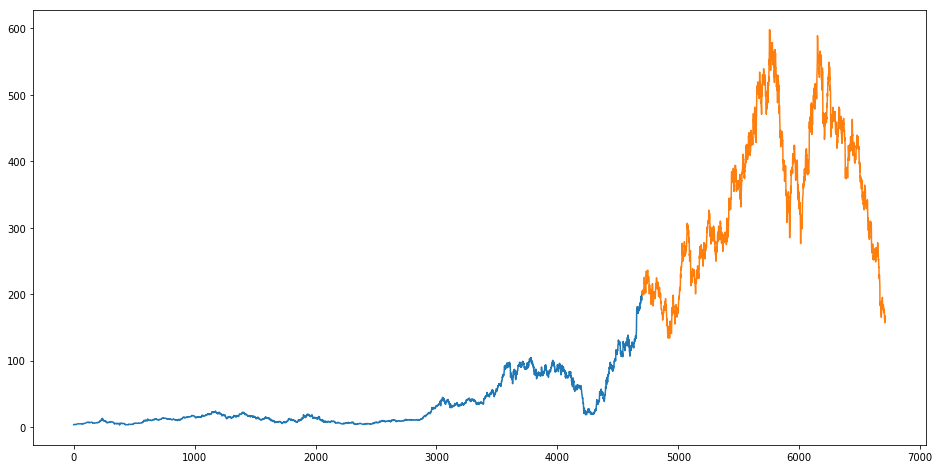In :
# Scaling the Data

from sklearn.preprocessing import StandardScaler
scaler = StandardScaler()
X_train_scaled = scaler.fit_transform(X_train)
X_test_scaled = scaler.transform(X_test)


## Machine Learning implementations¶

In :
# First we will use the simplest of them all - Linear Regression

from sklearn.linear_model import LinearRegression, LassoCV, RidgeCV, Lasso, Ridge
lr = LinearRegression()
lr.fit(X_train_scaled, y_train)

Out:
LinearRegression(copy_X=True, fit_intercept=True, n_jobs=None,
normalize=False)

For Cross Validation (CV) on Time Series data, we will use TimeSeries Split for CV.

Let's see Mean Absolute Error for our simplest model

In :
from sklearn.model_selection import TimeSeriesSplit
from sklearn.model_selection import cross_val_score
tscv = TimeSeriesSplit(n_splits=5)
cv = cross_val_score(lr, X_train_scaled, y_train, scoring = 'neg_mean_absolute_error', cv=tscv)
mae = cv.mean()*(-1)
mae

Out:
6573780029897.434

Oh Gosh!! Linear Regression failed miserably to predict the pattern. Lets try regularized linear models.

But before that, we will use the plotting module written in Topic 9 of the course to plot some nice graphs

In :
def plotModelResults(model, df_train, df_test, y_train, y_test, plot_intervals=False, plot_anomalies=False, scale=1.96, cv=tscv):
"""
Plots modelled vs fact values

model: fitted model

df_train, df_test: splitted featuresets

y_train, y_test: targets

plot_intervals: bool, if True, plot prediction intervals

scale: float, sets the width of the intervals

cv: cross validation method, needed for intervals

"""
# making predictions for test
prediction = model.predict(df_test)

plt.figure(figsize=(20, 7))
plt.plot(prediction, "g", label="prediction", linewidth=2.0)
plt.plot(y_test.values, label="actual", linewidth=2.0)

if plot_intervals:
# calculate cv scores
cv = cross_val_score(
model,
df_train,
y_train,
cv=cv,
scoring="neg_mean_squared_error"
)

# calculate cv error deviation
deviation = np.sqrt(cv.std())

# calculate lower and upper intervals
lower = prediction - (scale * deviation)
upper = prediction + (scale * deviation)

plt.plot(lower, "r--", label="upper bond / lower bond", alpha=0.5)
plt.plot(upper, "r--", alpha=0.5)

if plot_anomalies:
anomalies = np.array([np.NaN]*len(y_test))
anomalies[y_test<lower] = y_test[y_test<lower]
anomalies[y_test>upper] = y_test[y_test>upper]
plt.plot(anomalies, "o", markersize=10, label = "Anomalies")

# calculate overall quality on test set
mae  = mean_absolute_error(prediction, y_test)
mape = mean_absolute_percentage_error(prediction, y_test)
plt.title("MAE {}, MAPE {}%".format(round(mae), round(mape, 2)))
plt.legend(loc="best")
plt.grid(True);


Another plotting module for Coefficients

In :
def getCoefficients(model):
"""Returns sorted coefficient values of the model"""
coefs = pd.DataFrame(model.coef_, X_train.columns)
coefs.columns = ["coef"]
coefs["abs"] = coefs.coef.apply(np.abs)
return coefs.sort_values(by="abs", ascending=False).drop(["abs"], axis=1)

def plotCoefficients(model):
"""Plots sorted coefficient values of the model"""
coefs = getCoefficients(model)

plt.figure(figsize=(20, 7))
coefs.coef.plot(kind='bar')
plt.grid(True, axis='y')
plt.hlines(y=0, xmin=0, xmax=len(coefs), linestyles='dashed')
plt.show()


We will define a loss metric - namely - Mean Absolute Percentage Error which calculated Mean Absolute Error in percentage

In :
def mean_absolute_percentage_error(y_true, y_pred):
return np.mean(np.abs((y_true - y_pred) / y_true)) * 100


Let's see the plot for Linear Regression

In :
plotModelResults(lr, X_train_scaled, X_test_scaled, y_train, y_test, plot_intervals=True, plot_anomalies=True)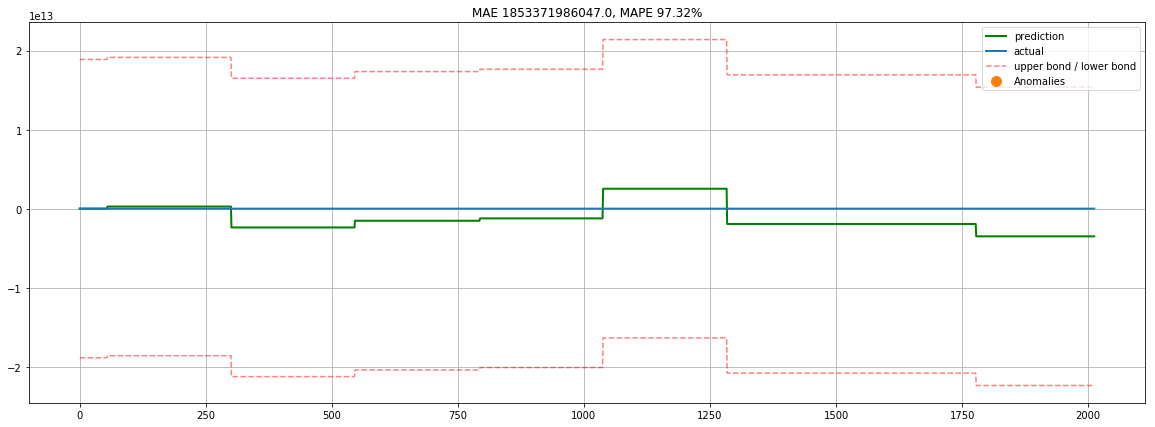This plot does not tell us much apart from the fact that our model has faired poorly in predicting the pattern.

Lets see the plot for coefficients

In :
plotCoefficients(lr)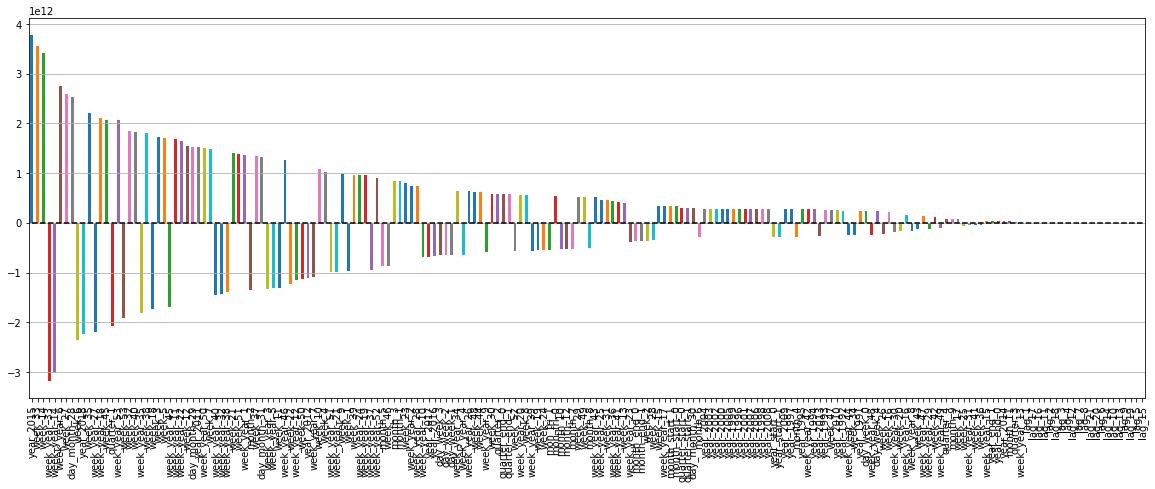Lets see the correlation matrix and Heat Map for the features

In :
import seaborn as sns
plt.figure(figsize=(15,10))
sns.heatmap(X_train.corr())

Out:
<matplotlib.axes._subplots.AxesSubplot at 0x11d73f60>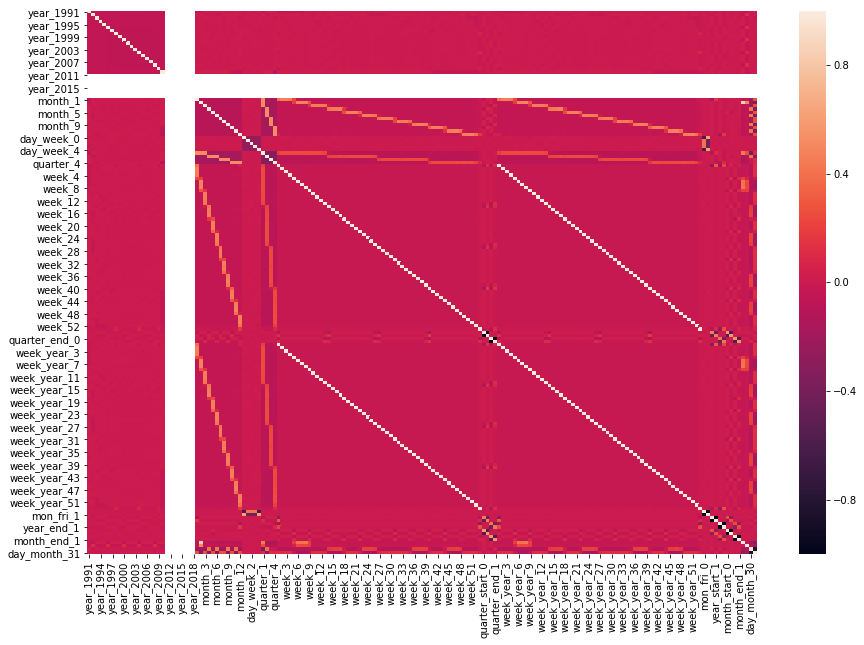Not much information can be derived from this Heat Map - only crucial information is prices in few years are completely uncorrelated.

Lets create our next model - Lasso Regression

In :
lasso = LassoCV(cv =tscv, max_iter=10000)
lasso.fit(X_train_scaled, y_train)

Out:
LassoCV(alphas=None, copy_X=True,
cv=TimeSeriesSplit(max_train_size=None, n_splits=5), eps=0.001,
fit_intercept=True, max_iter=10000, n_alphas=100, n_jobs=None,
normalize=False, positive=False, precompute='auto', random_state=None,
selection='cyclic', tol=0.0001, verbose=False)
In :
plotModelResults(lasso,
X_train_scaled,
X_test_scaled,
y_train,
y_test,
plot_intervals=True, plot_anomalies=True)
plotCoefficients(lasso)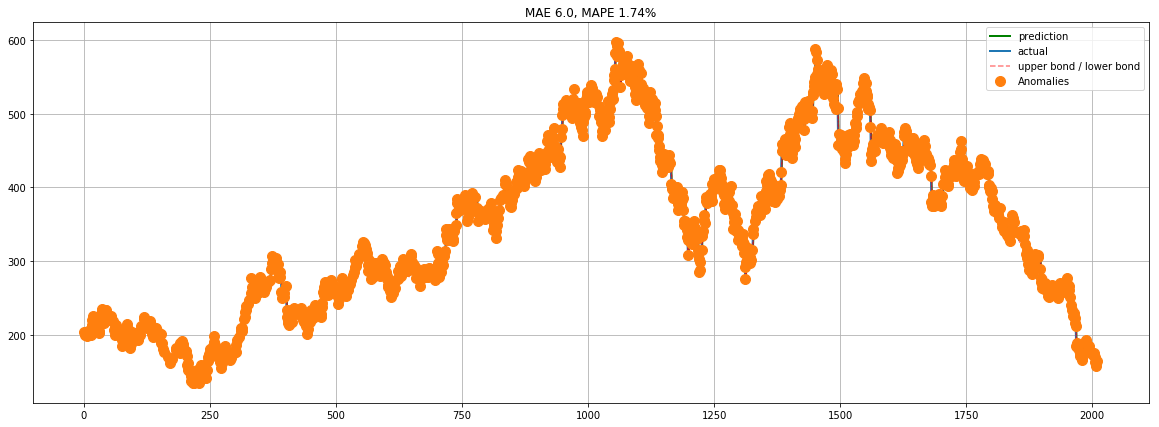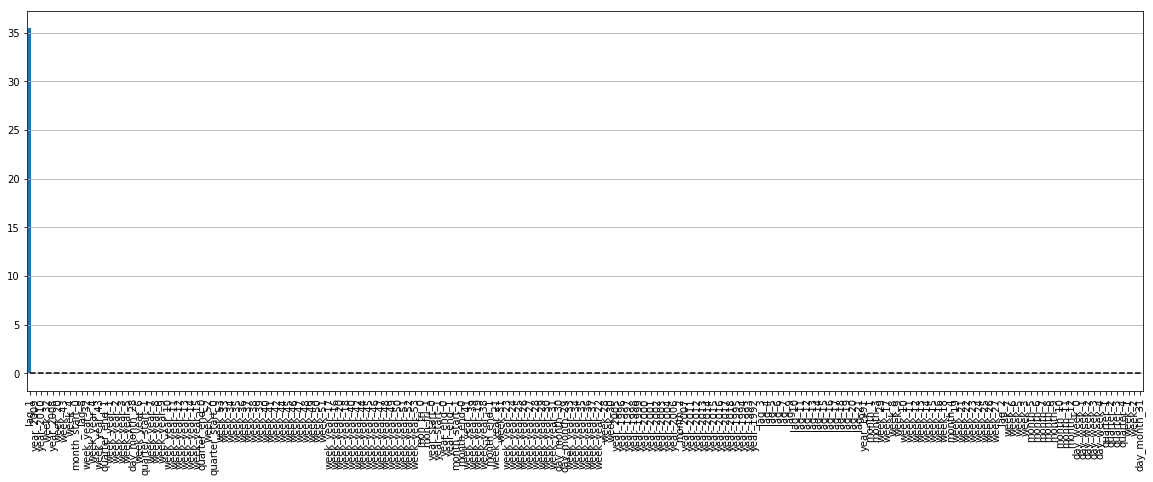In :
coef = getCoefficients(lasso)
np.count_nonzero(np.where(coef['coef']==0.000000))

Out:
181

Oh wow!

Around 181 features were of no value which are elimiated by the Lasso Regression

Let's see important features (Top10)

In :
coef.sort_values(by='coef', ascending=False).head(10)

Out:
coef
lag_1 3.550651e+01
year_2009 5.308872e-02
year_2010 3.609759e-02
week_32 3.299126e-02
lag_8 2.146558e-03
week_year_32 4.339372e-07
lag_11 0.000000e+00
year_1993 -0.000000e+00
year_1992 -0.000000e+00
lag_3 0.000000e+00

It turns out that Lag 1 is the most important feature

Lets see how close our predicted values are compared to actual values

In :
from sklearn.linear_model import Lasso
lasso = Lasso(max_iter=10000, random_state=17)

lasso.fit(X_train_scaled, y_train)
y_pred = lasso.predict(X_test_scaled)

columns = ['Close_actual', 'Close_pred']
df_pred_lasso = pd.DataFrame(columns = columns)

df_pred_lasso.Close_actual = y_test
df_pred_lasso.Close_pred = y_pred

In :
plt.figure(figsize=(15,8))
plt.plot(df_pred_lasso)
plt.plot(df_pred_lasso.Close_pred, "b--", label="prediction", linewidth=1.0)
plt.plot(df_pred_lasso.Close_actual, "r--", label="actual", linewidth=1)
plt.legend(loc="best")

Out:
<matplotlib.legend.Legend at 0x12bc6c18>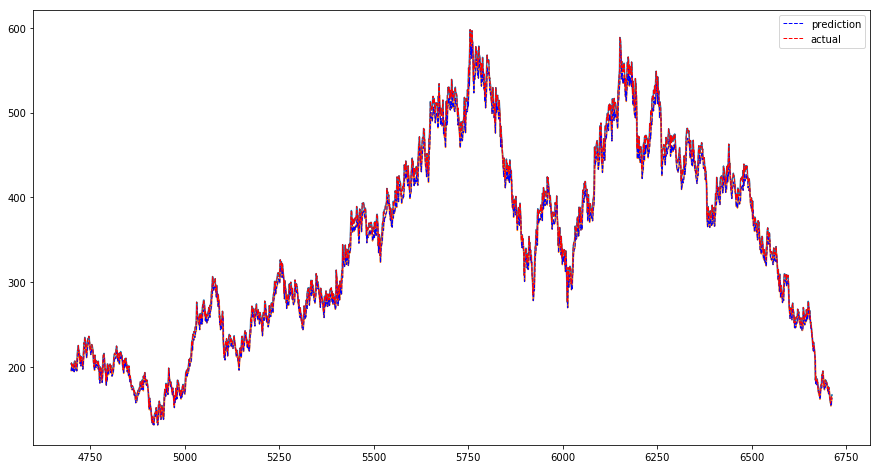In :
df_pred_lasso['diff'] = df_pred_lasso.Close_actual - df_pred_lasso.Close_pred
df_pred_lasso['perc_diff'] = ((df_pred_lasso['diff']) / (df_pred_lasso['Close_pred']))


Out:
Close_actual Close_pred diff perc_diff
4700 203.47 195.652330 7.81726 0.0399548
4701 204.664 198.977729 5.68589 0.0285755
4702 199.958 200.140080 -0.181813 -0.000908429
4703 202.19 195.559567 6.63034 0.0339045
4704 203.089 197.732002 5.35734 0.0270939
4705 200.235 198.607568 1.62781 0.00819613
4706 201.87 195.829330 6.04064 0.0308464
4707 198.714 197.420545 1.293 0.00654948
4708 205.079 194.347871 10.731 0.0552153
4709 206.652 200.544286 6.10794 0.0304568
4710 206.877 202.075922 4.80096 0.0237582
4711 206.281 202.294616 3.9861 0.0197044
4712 200.469 201.714272 -1.24551 -0.00617461
4713 202.475 196.056521 6.41832 0.0327371
4714 199.647 198.009370 1.63773 0.00827095
4715 205.061 195.256651 9.80469 0.0502144
4716 213.45 200.527248 12.9229 0.0644448
4717 219.538 208.693534 10.8449 0.0519655
4718 219.651 214.620201 5.03094 0.0234411
4719 225.29 214.729957 10.5601 0.0491784

Amazing!!

Lasso Regression has done a very nice job in predicting the adjusted closing price of this stock

We can also run PCA to eliminate more features and noises from the data

In :
from sklearn.decomposition import PCA
from sklearn.pipeline import make_pipeline

def plotPCA(pca):
"""
Plots accumulated percentage of explained variance by component

pca: fitted PCA object
"""
components = range(1, pca.n_components_ + 1)
variance = np.cumsum(np.round(pca.explained_variance_ratio_, decimals=4)*100)
plt.figure(figsize=(20, 10))
plt.bar(components, variance)

# additionally mark the level of 95% of explained variance
plt.hlines(y = 95, xmin=0, xmax=len(components), linestyles='dashed', colors='red')

plt.xlabel('PCA components')
plt.ylabel('variance')
plt.xticks(components)
plt.show()

In :
# Create PCA object: pca
pca = PCA()

# Train PCA on scaled data
pca = pca.fit(X_train_scaled)

# plot explained variance
plotPCA(pca)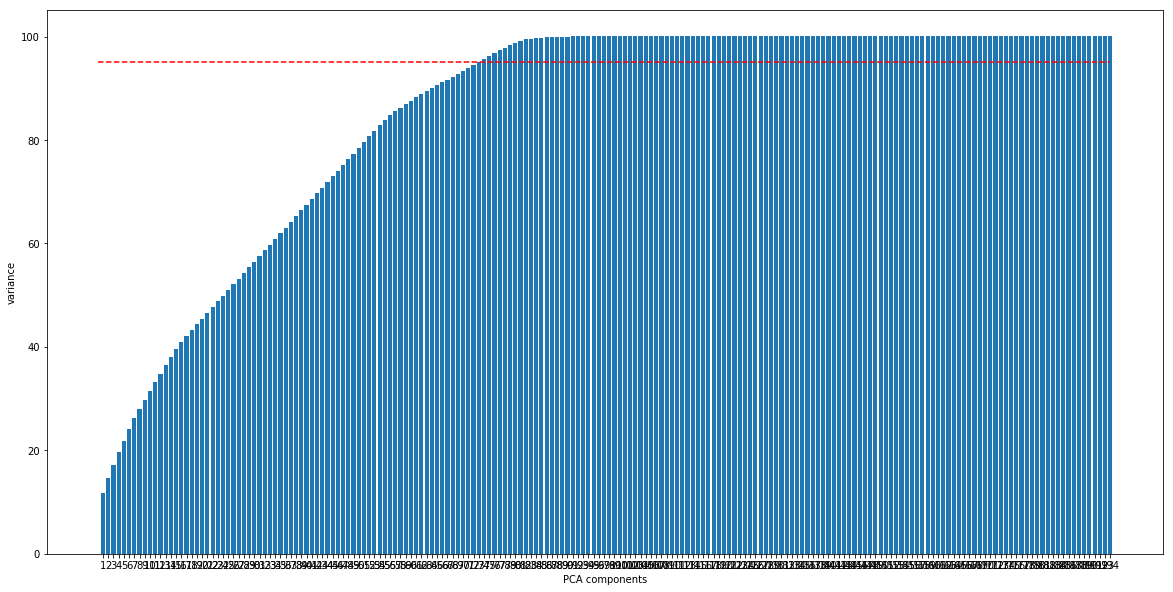In :
pca_comp = PCA(0.95).fit(X_train_scaled)
print('We need %d components to explain 95%% of variance'
% pca_comp.n_components_)

We need 73 components to explain 95% of variance


PCA needs only 73 components to explain the variance.

Lets fit and transform train and test data with these components

In :
pca = PCA(n_components=pca_comp.n_components).fit(X_train_scaled)

pca_features_train = pca.transform(X_train_scaled)
pca_features_test = pca.transform(X_test_scaled)


Lets run the Linear Regression model once again to see if there are any improvements since last time

In :
lr.fit(pca_features_train, y_train)

Out:
LinearRegression(copy_X=True, fit_intercept=True, n_jobs=None,
normalize=False)
In :
plotModelResults(lr, pca_features_train, pca_features_test, y_train, y_test, plot_intervals=True, plot_anomalies=True)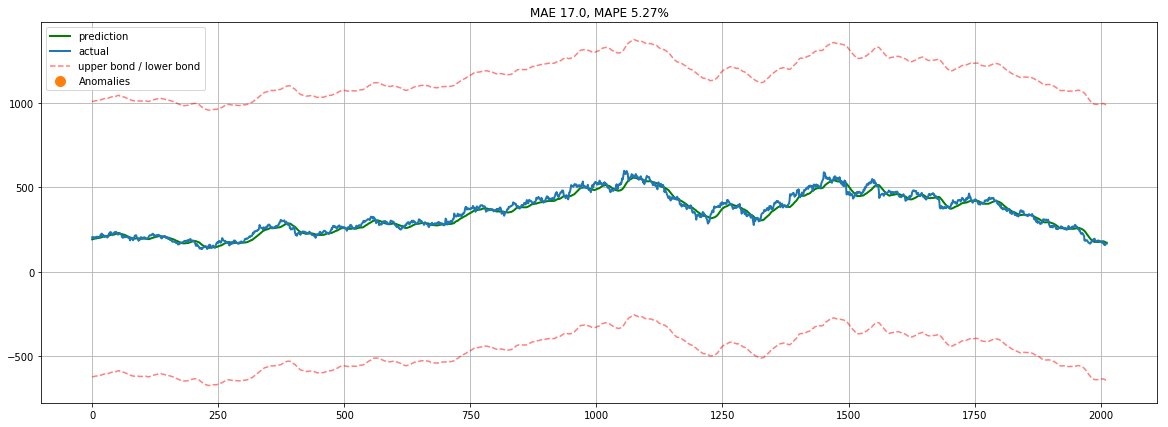Super!

PCA has resulted into an improvement in the linear regression model

Lets run another model - Ridge Regression and see how does it fare

In :
from sklearn.linear_model import Ridge
ridge = Ridge(max_iter=10000, random_state=17)

ridge.fit(X_train_scaled, y_train)
y_pred = ridge.predict(X_test_scaled)

columns = ['Close_actual', 'Close_pred']
df_pred_ridge = pd.DataFrame(columns = columns)

df_pred_ridge.Close_actual = y_test
df_pred_ridge.Close_pred = y_pred

In :
plt.figure(figsize=(15,8))
plt.plot(df_pred_ridge)
plt.plot(df_pred_ridge.Close_pred, "b--", label="prediction", linewidth=1.0)
plt.plot(df_pred_ridge.Close_actual, "r--", label="actual", linewidth=1.0)
plt.legend(loc="best")

Out:
<matplotlib.legend.Legend at 0x10f4eeb8>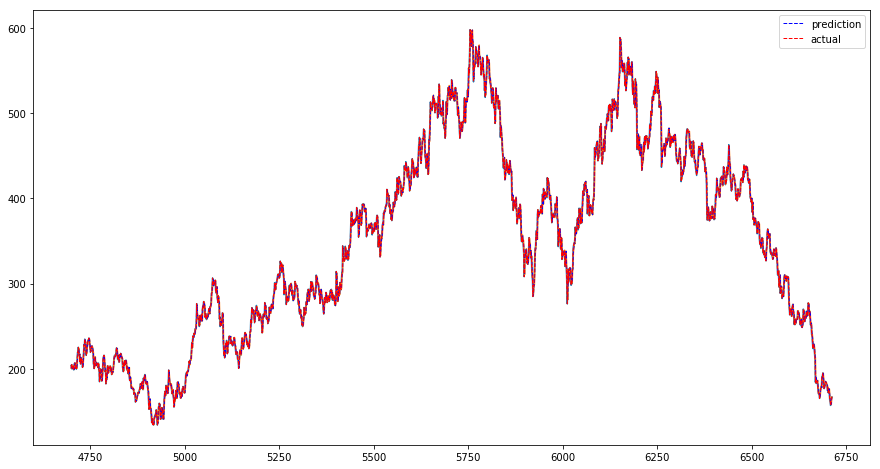In :
df_pred_ridge['diff'] = df_pred_ridge.Close_actual - df_pred_ridge.Close_pred
df_pred_ridge['perc_diff'] = ((df_pred_ridge['diff']) / (df_pred_ridge['Close_pred']))*100

Out:
Close_actual Close_pred diff perc_diff
4700 203.47 200.768340 2.70125 1.34546
4701 204.664 203.476201 1.18742 0.583567
4702 199.958 204.929404 -4.97114 -2.42578
4703 202.19 200.250048 1.93986 0.96872
4704 203.089 201.988105 1.10124 0.545198
4705 200.235 203.377847 -3.14247 -1.54514
4706 201.87 200.325602 1.54436 0.770926
4707 198.714 202.340981 -3.62743 -1.79273
4708 205.079 199.281304 5.79754 2.90922
4709 206.652 204.530661 2.12156 1.03728
4710 206.877 206.756599 0.120278 0.0581737
4711 206.281 206.377177 -0.0964613 -0.0467403
4712 200.469 206.263468 -5.7947 -2.80937
4713 202.475 201.571126 0.903712 0.448334
4714 199.647 202.145811 -2.49872 -1.2361
4715 205.061 200.438312 4.62303 2.30646
4716 213.45 204.856249 8.59395 4.19511
4717 219.538 213.327969 6.21042 2.91121
4718 219.651 219.360145 0.290993 0.132655
4719 225.29 219.161219 6.1288 2.79648

from sklearn.linear_model import RidgeCV